Courses

# Introduction (Part - 2) - Applications Of Viscous Flows Through Pipes Civil Engineering (CE) Notes | EduRev

## Mechanical Engineering : Introduction (Part - 2) - Applications Of Viscous Flows Through Pipes Civil Engineering (CE) Notes | EduRev

The document Introduction (Part - 2) - Applications Of Viscous Flows Through Pipes Civil Engineering (CE) Notes | EduRev is a part of the Mechanical Engineering Course Fluid Mechanics - Notes, Videos, MCQs & PPTs.
All you need of Mechanical Engineering at this link: Mechanical Engineering

Concept of Flow Potential and Flow Resistance

• Consider the flow of water from one reservoir to another as shown in Fig. 35.3. The two reservoirs A and B are maintained with constant levels of water. The difference between these two levels is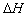as shown in the figure. Therefore water flows from reservoir to reservoir .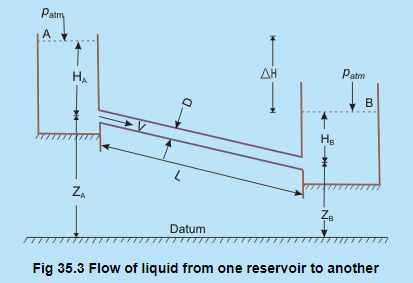• Application of Bernoulli's equation between two points and at the free surfaces in the two reservoirs giveswhere hf is the loss of head in the course of flow from to .

• Therefore, Eq. (35.10) states that under steady state, the head causing flow Δ H becomes equal to the total loss of head due to the flow.
• Considering the possible hydrodynamic losses, the total loss of head hf can be written in terms of its different components as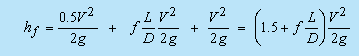35.11

Loss of heat at                 Friction loss in                           Exit loss to the
entry to the pipe                pipe over its                              reservoir B
from reservoir A                length L

where, is the average velocity of flow in the pipe.

(contd from previous...) Concept of Flow Potential and Flow Resistance

The velocity in the above equation is usually substituted in terms of flow rate , since, under steady state, the flow rate remains constant throughout the pipe even if its diameter changes. Therefore, replacing V in Eq. (35.11) as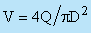we finally get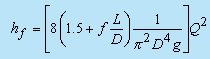(35.12)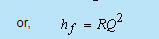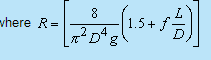(35.13)

The term is defined as the flow resistance .

In a situation where f becomes independent of Re, the flow resistance expressed by Eg. (35.13) becomes simply a function of the pipe geometry. With the help of Eq. (35.10), Eq. (35.12) can be written as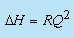( 35.14)

ΔH in Eq. (35.14) is the head causing the flow and is defined as the difference in flow potentials between A and B.

This equation is comparable to the voltage-current relationship in a purely resistive electrical circuit. In a purely resistive electrical circuit,  ΔV = Rl, where  ΔVis the voltage or electrical potential difference across a resistor whose resistance is R and the electrical current flowing through it is I

• The difference however is that while the voltage drop in an electrical circuit is linearly proportional to current, the difference in the flow potential in a fluid circuit is proportional to the square of the flow rate.
• Therefore, the fluid flow system as shown in Fig. 35.3 and described by Eq. (35.14) can be expressed by an equivalent electrical network system as shown in Fig. 35.4.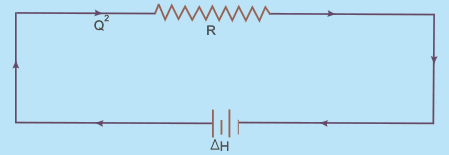Fig 35.4 Equivalent electrical network system for a simple pipe flow problem shown in Fig.35.3

Offer running on EduRev: Apply code STAYHOME200 to get INR 200 off on our premium plan EduRev Infinity!

## Fluid Mechanics - Notes, Videos, MCQs & PPTs

52 videos|76 docs|45 tests

,

,

,

,

,

,

,

,

,

,

,

,

,

,

,

,

,

,

,

,

,

;